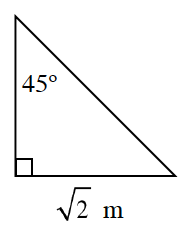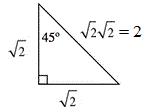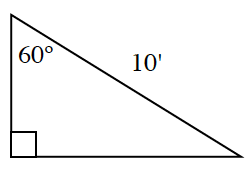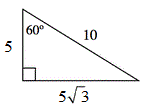### Home > INT2 > Chapter 6 > Lesson 6.1.1 > Problem6-6

6-6.

For each triangle below, use right triangle patterns to determine the missing side lengths. Then calculate the area and perimeter of each triangle.

1.Use a $45^{\circ}\text{-}45^{\circ}\text{-}90^{\circ}$ relationship to determine missing sides. Then calculate the area and perimeter of the triangle.$A = 1\text{ m}^2\text{, }P = 2 + 2\sqrt{2}\text{ m}$

1.Use a $30^{\circ}\text{-}60^{\circ}\text{-}90^{\circ}$ relationship to determine the missing sides. Then calculate the area and perimeter of the triangle.$A = \frac{25\sqrt{3}}{2}\approx21.7\text{ ft}^2\text{, }P\approx15+5\sqrt{3}\approx23.7\text{ ft}$